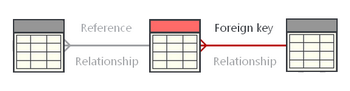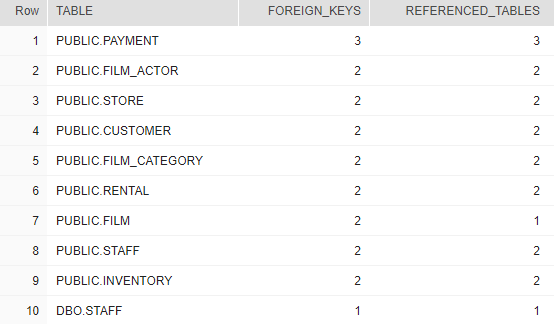## List tables with most foreign keys in Snowflake

Article for:

Query below lists tables with their number of foreign keys and number of tables they refer to.## Query

``````select fk_tco.table_schema || '.' || fk_tco.table_name as "TABLE",
count(*) as foreign_keys,
count(distinct pk_tco.table_name) as referenced_tables
from information_schema.referential_constraints rco
join information_schema.table_constraints fk_tco
on rco.constraint_name = fk_tco.constraint_name
and rco.constraint_schema = fk_tco.table_schema
join information_schema.table_constraints pk_tco
on rco.unique_constraint_name = pk_tco.constraint_name
and rco.unique_constraint_schema = pk_tco.table_schema
group by "TABLE"
order by count(*) desc;
``````

## Columns

• table - table name preceded by schema name
• foreign_keys - number of references (FKs)
• referenced_tables - number of referenced tables. This may be other value than number of references, because multiple FKs can refer to one table

## Rows

• One row represents one table
• Scope of rows: tables that reference other tables
• Ordered by number of foreign keys descending

## Sample results